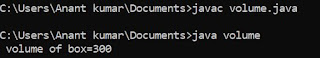## Wednesday, 8 August 2018

### Program To Find Factorial Of Any Number in " C" Language

/* factorial of a number */
# include <stdio.h>
void main ()
{
int a ;
fact = 1;
clrscr () ;
printf ("\n enter a number:") ;
scanf ("%d", & a) ;
while ( a!=0 )
{
fact = fact*a ;
a-- ;
}
printf ("\n factorial of %d =%d", a,fact) ;
getch () ;
}

### Output in c :

Enter a number : 4
factorial : 4 * 3 * 2 * 1 = 24
Enter a number : 6
factorial : 6 * 5 * 4 * 3 * 2 * 1 = 720

ALGORITHM :  TO FIND FACTORIAL OF A NUMBER INPUT FROM USER IN C

This is a program for calculation of factorial of a number input from user in c.

STEP 1. START.
STEP 2. SET FACT = 1.
STEP 3. INPUT A NUMBER SAY A.
STEP 4. { CHECK FOR CONDITION }
A ! = 0.
{ HERE A ! = 0 BECAUSE FACTORIAL IS ALWAYS MULTIPLE OF MAXIMUM OF THE GIVEN NUMBER TO ONE}
STEP 5. FACT = FACT * A.
STEP 6. A = A - 1.
STEP 7. PRINT VALUE OF FACT.
STEP 8. STOP.